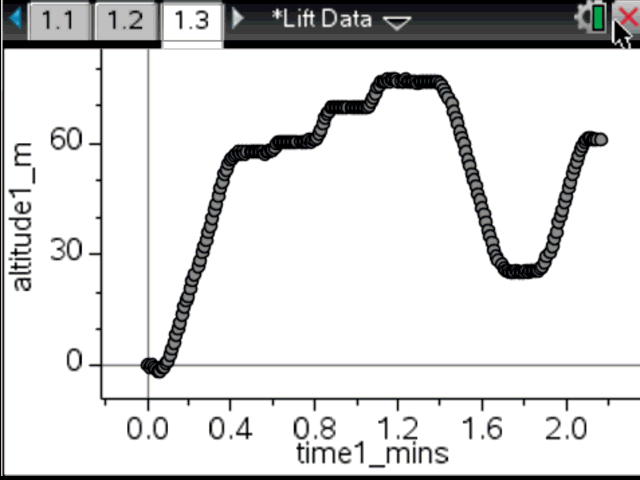# Activities

•• ##### Subject Area

• Standard: National Curriculum 7-10: Number and Algebra: Linear and non-Linear Relationships
• Standard: National Curriculum 7-10: Number and Algebra: Number and place value

• ##### AuthorMiddle

90 Minutes

• ##### Device
•TI-Nspire™ CX
•TI-Nspire™ CX CAS
• ##### Software

TI-Nspire™
TI-Nspire™ CAS

3.2

## Lift Data#### Activity Overview

Students will use authentic data to graph and analyse the altitude of two different lifts over a period of time in order to determine travel time, speed and the number of floors visited.

#### Objectives

• ACMNA208 – Solve problems involving direct proportion. Explore the relationship between graphs and equations corresponding to simple rate problems
• Students learn to use a line of best fit to estimate the gradient and speed of a lift. They also learn to use the gradient formula to determine the slope of a line between two points.

• Acceleration
• Velocity
• Speed
• Distance
• Slope